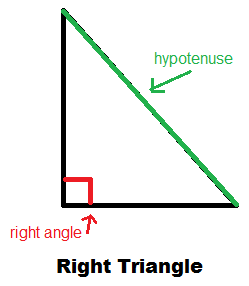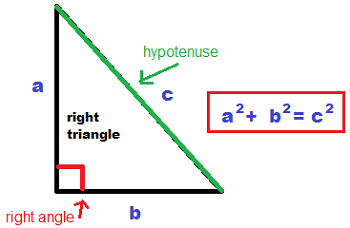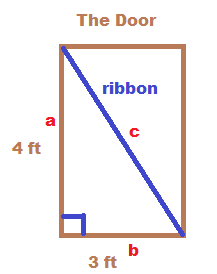# Pythagorean Theorem Lesson for Kids: Definition & Examples

Instructor
Bethany Calderwood

Bethany is a certified Special Education and Elementary teacher with 11 years experience teaching Special Education from grades PK through 5. She has a Bachelor's degree in Special Education, Elementary Education, and English from Gordon College and a Master's degree in Special Education from Salem State University.

Expert Contributor
Laura Pennington

Laura received her Master's degree in Pure Mathematics from Michigan State University, and her Bachelor's degree in Mathematics from Grand Valley State University. She has 20 years of experience teaching collegiate mathematics at various institutions.

An important part of geometry is knowing how to measure shapes. The Pythagorean Theorem is used in the measurement of triangles. In this lesson, you will learn the Pythagorean Theorem and how to use it. Then you will practice on a triangle of your own. Updated: 10/06/2020

## Relationships

Scientists and mathematicians like to study relationships. They study how a plant or animal relates to its environment, and how numbers relate to nature and music. When a specific relationship is found over and over, they give it a name and share the information. One example is the Pythagorean Theorem, which explains the relationship between the sides of a right triangle.

## The Anatomy of a Triangle

Before you can learn about the Pythagorean Theorem, you need to know a few terms.### Right Triangle

A right triangle has one ninety-degree angle inside the triangle, which is called a right angle. Mathematicians often mark the right angle with a box. On the diagram of the triangle, the box is drawn in red.

### Hypotenuse

In a right triangle, the hypotenuse is the side directly across from the right angle. It is the only side of the triangle that is not a part of the right angle. On the diagram, the hypotenuse is green.

### Exponents

An exponent appears slightly above and to the right of a number, like this: 32. A number with an exponent is actually a multiplication sentence, and the exponent indicates how many times that number appears in the multiplication sentence. So this example, 32, means 3 x 3. An exponent of 2 is read ''to the second power'' or ''squared.''

## The Pythagorean Theorem

The Pythagorean Theorem states the relationship between the sides of a right triangle, when c stands for the hypotenuse and a and b are the sides forming the right angle. The formula is:

a2 + b2 = c2

It is read ''a-squared plus b-squared equals c-squared.''## Using the Pythagorean Theorem

Leila's school is having a door-decorating contest. Leila's class wants to use ribbon to divide their door into two triangles. They know the length of each side of the door. To find out how long the ribbon needs to be, they can use the Pythagorean Theorem.To unlock this lesson you must be a Study.com Member.

## Real-World Applications of the Pythagorean Theorem:

### Reminders:

• The Pythagorean Theorem applies to right triangles, where a right triangle is a triangle with a right, or 90-degree, angle.
• The hypotenuse of a right triangle is the side opposite the 90-degree angle in the triangle.
• The Pythagorean Theorem states that if a right triangle has two sides with lengths a and b, and a hypotenuse of length c, then a^2 + b^2 = c^2.

### Real-World Applications:

1. Suppose a support beam is to be built so that it runs between the floor and a wall. The distance up the wall that the beam reaches is 8 feet, and the other end of the beam is on the floor 6 feet from the wall. What should the length of the support beam be?
2. A surveyor is trying to determine the distance across a pond without having to actually get in the pond to measure it. Because of how the pond is shaped, they are able to create a right triangle with a hypotenuse being the distance across the pond, and the other two sides are on land. The surveyor measures the two sides on land to be 24 feet and 32 feet. What is the distance across the pond?

### Solutions and Explanations:

1. In this scenario, the beam, the floor, and the wall create a right triangle, with the beam being the hypotenuse, and the other two sides having lengths 6 feet and 8 feet. Thus, the Pythagorean Theorem gives that 6^2 + 8^2 = c^2, where c is the length of the support beam. Simplifying the left-hand side of this equation gives 100 = c^2. Since 10 times 10 is 100, we know that c = 10, so the support beam should be 10 feet.
2. In this problem, the distance across the pond is the hypotenuse of a right triangle with sides of length 24 feet and 32 feet, so the Pythagorean Theorem gives that 24^2 + 32^2 = c^2, with c being the distance across the pond. Simplifying the left-hand side of this equation gives 1,600 = c^2. Since 40 times 40 equals 1,600, we have that c = 40. Therefore, the distance across the pond is 40 feet.

### Register to view this lesson

Are you a student or a teacher?